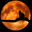Related Tags

python
pandas
data analysis
community creatorNeko Yan

When we load a file into a pandas DataFrame object, we may find that it consumes more memory than we thought. There are two reasons for this:

• Some unnecessary fields are also loaded.
• The default field types are the most memory-consuming, e.g., int64 is the default type for integer field.

The first method is to load some fields, but not all of them. For example, we are trying to load a CSV file by read_csv. By default, it loads all fields. However, read_csv allows you to pass the column names to usecols, which means only those columns in this list would be loaded.

import numpy as np
import pandas as pd
import os

# At first, we create a dataset with 20000 rows and 10 columns.
# Meanwhile, we assign 10 column names for these 10 columns.
d = np.random.randint(0, 20, size=(20000, 10))
df = pd.DataFrame(d,
columns=["a", "b", "c", "d", "e", "f", "g", "h", "i", "j"])

# Export this dataset to a csv file with sep=\t and without index.
df.to_csv("output/raw.csv", sep='\t', index=False)

# At first, we load all columns from this file.
# Then print the information of this dataframe object.
print(full_df.info())

print("----------------------------------------------------------------")

# Then, we load this file again, but with only 3 fields.
# Then print the information of this dataframe object again.
less_df = pd.read_csv("output/raw.csv", sep='\t', usecols=["a", "b", "c"])
print(less_df.info())

os.remove("output/raw.csv")
Load file with part of fields

As you can see from the output of this code widget:

• The memory usage of the first DataFrame object (output of line 17) is 1.5MB.

• The memory usage of the second DataFrame object (output of line 24) is 46BKB, which is about a third.

Notice: Because of the limitation of this site, I can’t create a dataset with too much size. However, from the output of the last example, choosing selected columns can reduce memory usage greatly, if your dataset is h​uge.

## Reduce memory by specifying column types

The second method is to specify the type of each column. The read_csv allows you to pass a dict (the key is the column name, value is the type) to dtype. In this example, all data value is between 0 and 20, however, the default type is int64a signed 64-bit integer. Obviously, for such data, the uint8an unsigned 8-bit integer type is sufficient.

import numpy as np
import pandas as pd
import os

# At first, we create a dataset with 20000 rows and 10 columns.
# Meanwhile, we assign 10 column names for these 10 columns.
d = np.random.randint(0, 20, size=(20000, 10))
df = pd.DataFrame(d,
columns=["a", "b", "c", "d", "e", "f", "g", "h", "i", "j"])

# Export this dataset to a csv file with sep=\t and without index.
df.to_csv("output/raw.csv", sep='\t', index=False)

# At first, we load all columns from this file.
# Then print the information of this dataframe object.
print(full_df.info())

print("----------------------------------------------------------------")

# Specify data type for each column.
# The key is column name, value is data type
dtype = {
"a": 'uint8',
"b": 'uint8',
"c": 'uint8',
"d": 'uint8',
"e": 'uint8',
"f": 'uint8',
"9": 'uint8',
"h": 'uint8',
"i": 'uint8',
"j": 'uint8'
}

# Then, we load this file again, but specify the data type for each column.
# Then print the information of this dataframe object again.
print(less_df.info())

os.remove("output/raw.csv")
Load file and specify data type for each column

As you can see from the output of this code widget:

• The memory usage of the first DataFrame object (output of line 17) is 1.5MB.

• The memory usage of the second DataFrame object (output of line 38) is 332KB, which is about a fifth.

RELATED TAGS

python
pandas
data analysis
community creator

CONTRIBUTORNeko Yan
RELATED COURSES

View all Courses

Keep Exploring

Learn in-demand tech skills in half the time